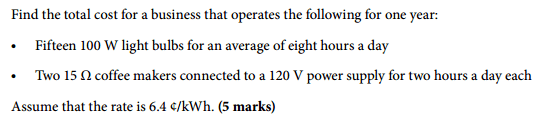# Calculating the cost of electricity

## Homework Statement## Homework Equations

Cost = rate x energy consumption (kWh)
Energy consumption = power (kW) x total time (hrs)

## The Attempt at a Solution

so here are my answers, can someone please verify if these are correct, thanks!

a)

To calculate power:
There are fifteen 100 W lightbulbs, so to calculate the total power, i would do the following:
P = 15 x 100 W = 1500 W = 1.5 kW

To calculate total time:
8 hrs (per day) x 365 days (since the business operates the following for one year) = 2920 hrs

To calculate electrical energy used in kWh:
E = P x t
E = (1.5 kW) x (2920 hrs)
E = 4380 kWh

To calculate total cost:

I already know the rate: 6.4 cents /kWh

cost = rate x total energy consumption (kWh)
cost = (6.4 cents /kWh) x (4380 kWh)
cost = 28032 cents = $280.32 b) To calculate power: P = V^2 / R P = (120 V)^2 / 15 ohms P = 960 Watts (multiply this by 2, since there are 2 coffee makers) P = 960 watts x 2 P = 1920 Watts = 1.92 kW To calculate total time: total time = 4 hrs (since both are used for two hours each) x 365 days (since the business operates for one year) total time = 1460 hrs To calculate energy consumption in kWh: Energy = power (kW) x total time (hrs) Energy = (1.92 kW) x (1460 hrs) Energy = 2803.2 kWh To calculate total cost: cost = rate x energy consumption cost = (6.4 cents/kWh) x (2803.2 kWh) cost = 17940.48 cents =$179.4048

#### Attachments

gneill
Mentor
For your calculation of the cost of running the coffee makers you have accounted for the them running at the same time twice: once when you found the total power: P = 960 W x 2, and again when you doubled the total time.

so how would i calcualte the power for part b)
do i still use the same formula P = V^2 / R
instead of doubling 960, do i double 15 Ohms, and then calculate, so like this:
P = (120 V)^2 / 30 Ohms = 480 W = 0.48 kW
in the question, it was mentioned that the two coffee makers are on for two hours a day each, does that mean that the coffee makers are on at the same time, so for 2 hrs a day, or are they in use during seperate times of the day, so 2 hrs + 2 hrs = 4 hrs.
so im assuming that the total time is just 2 hrs x 365 days ( since the business operates for one year) = 730 hrs
im really confused :P

gneill
Mentor
I think you can assume that they both run for 2 hrs each day. Presuming that they run at the same time is the straightforward way to proceed, but you can break it down further if you want. You might, for example pretend they are separate entities ("Coffee makers A and B") that each run for two hours. But it's simpler to combine them (like the light bulbs) and use the total power that they use.

So, bottom line: They use 960 W for 4 hours if they run at separate times during the day or 1920 W for 2 hours if they run in parallel. Both amount to the same amount of usage.

ok, so here is my final answer for part b, please confirm is this is correct, thanks.

To calculate power:
P = V^2 / R
P = (120 V)^2 / 15 ohms
P = 960 Watts (multiply this by 2, since there are 2 coffee makers)
P = 960 watts x 2
P = 1920 Watts = 1.92 kW

To calculate total time:
total time = 2 hrs x 365 days (since the business operates for one year)
total time = 730 hrs

To calculate energy consumption in kWh:
Energy = power (kW) x total time (hrs)
Energy = (1.92 kW) x (730 hrs)
Energy = 1401.6 kWh

To calculate total cost:
cost = rate x energy consumption
cost = (6.4 cents/kWh) x 1401.6 kWh
cost = 8970. 24 cents = \$89.7024

gneill
Mentor
Looks good!

ok thanks for the clarification :)